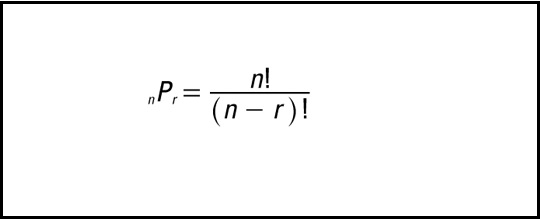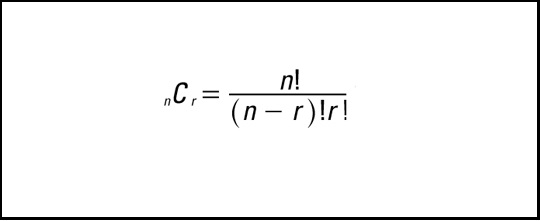# How to calculate combination and permutation in C++?

Combination and permutation are a part of Combinatorics. Permutation is the different arrangements that a set of elements can make if the elements are taken one at a time, some at a time or all at a time. Combination is is the different ways of selecting elements if the elements are taken one at a time, some at a time or all at a time.

Number of permutations when there are total n elements and r elements need to be arranged.Number of combinations when there are total n elements and r elements need to be selected.A program that calculates combination and permutation in C++ is given as follows.

## Example

#include <iostream>
using namespace std;
int fact(int n) {
if (n == 0 || n == 1)
return 1;
else
return n * fact(n - 1);
}
int main() {
int n, r, comb, per;
cout<<"Enter n : ";
cin>>n;
cout<<"\nEnter r : ";
cin>>r;
comb = fact(n) / (fact(r) * fact(n-r));
cout << "\nCombination : " << comb;
per = fact(n) / fact(n-r);
cout << "\nPermutation : " << per;
return 0;
}

## Output

The output of the above program is as follows.

Enter n : 5
Enter r : 3
Combination : 10
Permutation : 60